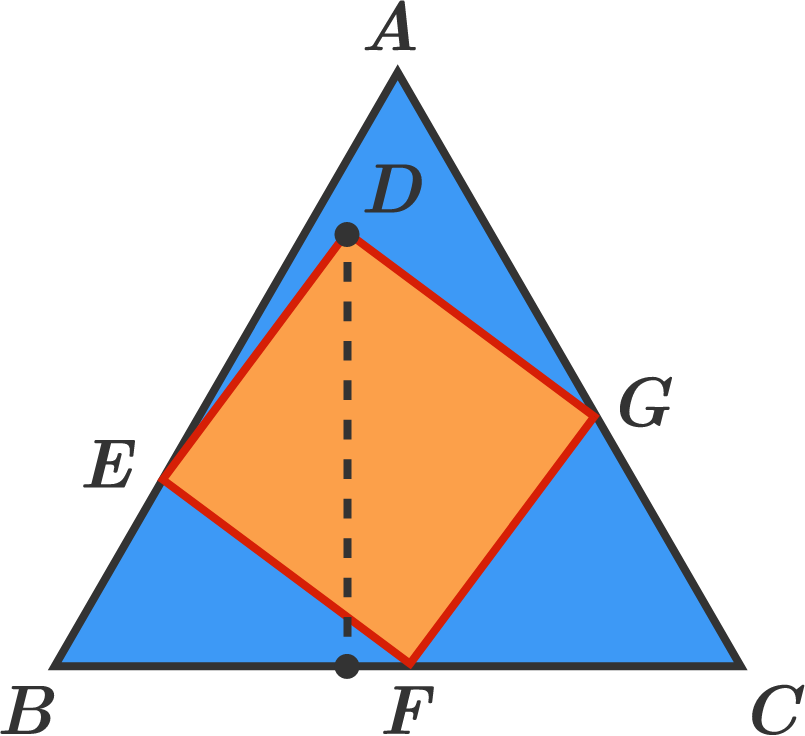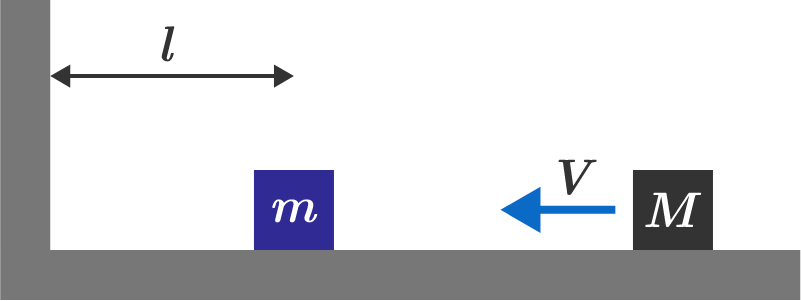# Problems of the Week

Contribute a problem

$\phi(n)$ is the number of positive integers less than $n$ that are relatively prime to $n.$

Find the remainder when $\phi\big(2^{2018} + 1\big)$ is divided by 4036.

Bonus: Generalize for the remainder when $\phi(2^n+1)$ is divided by $2n.$

$ABC$ is an equilateral triangle with side length 1. Square $DEFG$ is placed in triangle $ABC$ such that points $E,$ $F,$ and $G$ are on segments $AB,$ $BC,$ and $CA,$ respectively.

What is the length of the perpendicular from point $D$ to segment $BC?$$\begin{pmatrix}x & y & y \\ y & x & y \\ y & y & z \end{pmatrix}\begin{pmatrix}a \\ b \\ c \end{pmatrix} = \begin{pmatrix}a' \\ b' \\ c' \end{pmatrix}$ If the above equation holds true, then there exist coprime positive integers $x, y, z$ such that any Pythagorean triple $(a, b, c)$ produces another Pythagorean triple $\big(a', b', c'\big).$

Find the sum of such $x, y,$ and $z.$

$\int_{1}^{e}\ln (x)+\frac{1}{2}\left ( \ln (x)+\frac{1}{3}\Big ( \ln (x)+\tfrac{1}{4}\big( \ln (x)+\tfrac{1}{5} {\small(\cdots)}\, \big) \Big ) \right )\, dx = \, ?$

A block of mass $m$ sits on a horizontal, frictionless surface some distance $\ell$ from a wall. Another block of mass $M$ collides from the right with velocity $V.$ Depending on that ratio $x =\frac Mm,$ the blocks will collide a total of $n$ times.

Find $\displaystyle \lim_{x\rightarrow \infty} \frac{n}{\sqrt{x}}.$Assume that all collisions are elastic and that the motion is in 1-dimension.

×